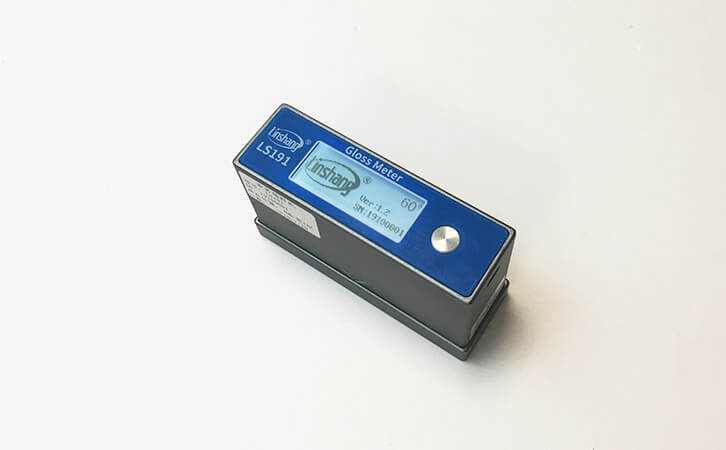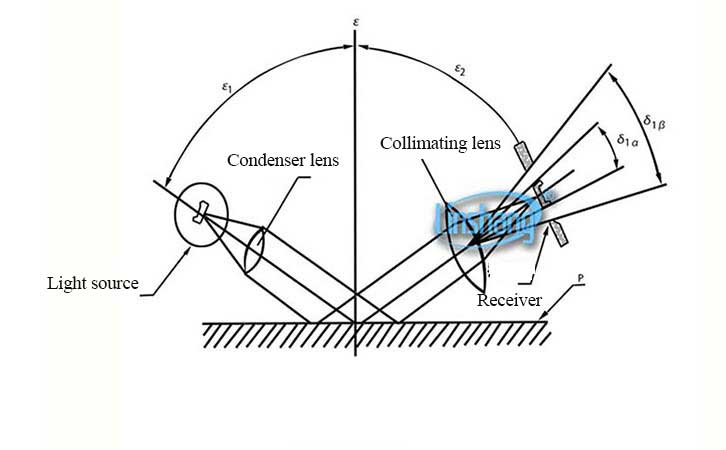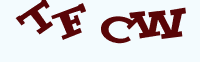# Difference Between 60 Degree Gloss Meter and Multi-angle Gloss Meter

Time:2019/10/12 11:12:00 Browse:652

The biggest difference between a single-angle gloss meter and a multi-angle gloss meter is that a single-angle gloss meter can measure only one angle (mostly 60 degree), while a multi-angle gloss meter can measure multiple angles by 20 degree. 45 degree, 85 degree, etc.

A 60 degree angle gloss meter is usually used to determine whether an object is high gloss or low gloss, based on 10-70 gloss units. A small angle gloss meter is used when the gloss value is higher than 70 GU. A high angle gloss meter is used when the gloss value is lower than 10 GU.Below we will understand the difference between a single-angle gloss meter and a multi-angle gloss meter from the internal structure of the gloss meter.

1. First let's take a look at what is a normal. The bisector of a corner is often called the normal. What we usually call a 60 degree angle gloss meter is a gloss meter that is at an angle of 60 degrees to the normal. Inside the gloss meter, there will be a convex mirror located at a position 60 degrees from the normal. It gathers the light on the horizontal surface. The internal light components of the instrument convert the light energy into electrical energy and display it on the meter.2. The multi-angle gloss meter is internally equipped with a number of convex mirrors, such as multi-angle gloss meters that can measure 20 degree, 45 degree and 80 degree. Three convex mirrors are installed inside and the three convex mirrors are respectively at an angle of 20 degree, a angle of 45 degree and an angle of 80 degree with the normal. When we want to measure a 20 degree angle, the instrument automatically uses a 20 degree angle lens. When we want to measure other angles, the instrument automatically uses the lens at other angles. For the multi-angle gloss meter on the market at this stage, it is basically an independent lens. Of course, due to the difference in use and internal structure, the price of the multi-angle gloss meter and the single-angle gloss meter are also different.Click image refresh captcha